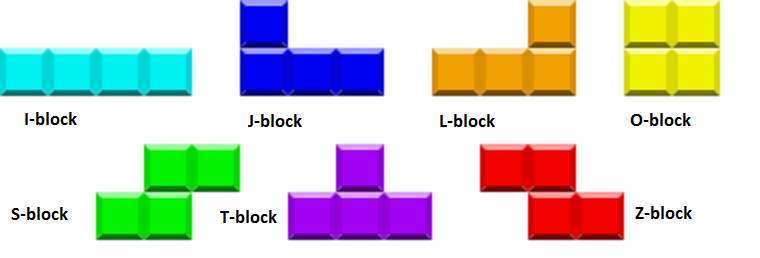top of page
Search
• KJ Ha

# Nature of Code: Genetic Algorithm Design Exercise

### Scenario

When I heard the concept of genetic algorithm for the first time, I automatically thought it is perfect for games, like in the smart rocket example. To keep it simple and sweet, I propose the Tetris game scenario.

I first wondered whether the population in this scenario should be the shape of blocks or their movements. But soon I figured, it makes more sense to set the population to be the movements of each block when considering the objective of the game.

### Phenotype and GenotypeDifferent types of tetrominoes

In short, the main factor that needs to be evolving is the movement pattern of each block type. For example, while rotating the O-block is just a waste of time, rotating J-block can give us different results each time. However, to achieve the optimal result, many other factors need to be taken into consideration.

Pictures showing very different phases of the game.

Here are some factors that affect the final result of the game.1. The total height of each vertical line (ex: 4+4+4+4+5+6+4+4+4+2=41) => need to be minimized2. The total number of cleared lines. => need to be maximized3. The total number of holes (ex: 3) => need to be minimized4. The total number of blocks above the holes (ex: 4) => need to be minimized5. The standard deviation of heights (ex: 0.94339811320566)6. The number of faces of a descending block that will touch the ground. (ex: 2)7. The number of faces of a descending block that will touch the wall. (ex: 2)

### Fitness Function

The objective of the Tetris game is to get the highest score by completing as many horizontal lines of blocks within the longest survival time.

### Mutation and Crossover

1. Run the game multiple times with random values for seven factors that affect the final result. (Now, I only can think of seven, but there could be more factors.)

2. Take high-scoring values of the factors.

3. Mix high-scoring values across different games until achieving the highest score.

## Recent Posts

See All

Link to video recording: https://youtu.be/_n7wWtambYc For the final, I would like to continue working on the mid-semester exercise. (link to mid-semester exercise: https://editor.p5js.org/kh1785/sketc

Link to code: https://editor.p5js.org/kh1785/sketches/tT1Cj0ZRp9 For this week's assignment, I've created a sketch inspired by one of the video examples, the Game of Life. A lot was taken directly fro

bottom of page